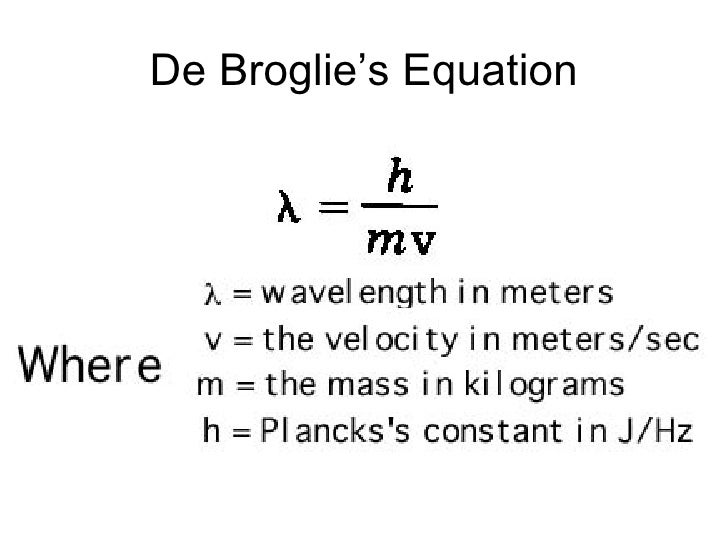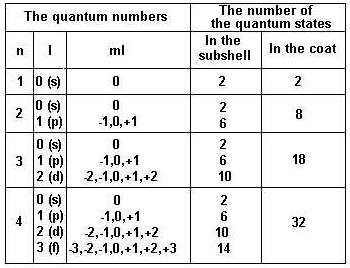Resources:

Have you found videos, websites, or explanations that helped you understand this chapter? Let us know and we'll add them to "Resources" part of this page for other students to use.

Use CTRL+ F to find all questions. All answers are available

Question 1

Gamma rays are electromagnetic radiation of very short wavelength emitted by the nuclei of radioactive elements. A certain radioactive element emits a gamma ray with a frequency of 1.51 x 1020 Hz. Express the wavelength of this radiation in picometers. (1Hz = 1 s-1 and 1 picometer = 1 x 10-12 m)Explanation

λ= c / f

c= 3E8 m/s

λ = (3E8 m/s)/  (1.51 E 20) = 1.987 E-12 = 1.987 pm

Question 2

The photoelectric effect for a certain alloy has a threshold frequency of 9.20 x 1014 Hz. For light of this frequency, find the energy of one mole of photons (in kJ).

Hint given in feedbackExplanation

E = h x f x (Avogadro number) / 1000

E = (6.63E-34 ) (9.20E14) (6.02E23 photons) / 1000 = 367

Question 3

Electrons are ejected from sodium metal by any light that has a wavelength shorter than 544 nm. What is the kinetic energy of an electron (in J) that is ejected from sodium by light with a wavelength of 471 nm?Explanation

E = (hc/ λ) - (hc/λ)

E = [ (3E8 x 6.63E-34)/ (544 E-9) ] - [ (3E8 x 6.63E-34)/ (213 E-9) ]

E = 5.68E-19

Question 4

The charge of an electron is -1.602 x 10-19 C. For the photoelectric effect, how many microamperes (1 A = 1 C/s) of electrical current are produced when a metal is bombarded by 6.60x1013 photons each second? Assume that each photon has sufficient energy to eject one electron. (Note currents are reported as positive values.)

Hint given in feedbackQuestion 5

What is the wavelength (in nm) of the line in the spectrum of the hydrogen atom that arises from the transition of the electron from the orbital with n =7 to the orbital with n = 3?

Note, the line corresponds to the energy of the photon that is emitted in the transition.ExplanationE = -2.18E-18 ( 1/9 -1/49) =1.977E-19(6.63E-34)(3E8)/ 1.977E-19  = 1009

Question 6

Find the de Broglie wavelength (in nm) associated with an electron that is moving with a velocity of 4890 km/s. The electron rest mass is 9.11 x 10-31kg.

Note, electrons having this speed would need to be treated as waves in atoms because the wavelength is on the order of the size of atoms.Explanationλ = (6.63E-34)/ (4890E3)(9.11E-31) = .1487 nm

Question 7

A baseball weighs 220 g. Top speed for a professional pitcher is about 100 mph when he throws a fast ball. Find the de Broglie wavelength (in nm) associated with a baseball that is moving with a velocity of 45 mph.

Hint given in feedbackExplanationλ = (6.63E-34)/ (.220kg)(20.1) = 1.499E-34 / 1E-9 = 1.5E-25 nm

Question 8

In each statement below, a set of quantum numbers is given. Check the allowed combinations of n, l, ml, and ms. (n = principle quantum number, l = angular momentum q.n., ml = magnetic q.n., and ms = electron spin q.n.)

Select one or more:ExplanationQuestion 9

In each statement below, a set of quantum numbers is given. Match the 4d subshell with the correct allowed combinations of n, l, ml, and ms.

Select one:ExplanationQuestion 10

In each statement below, a set of quantum numbers is given. Match the 2s subshell with the correct allowed combinations of n, l, ml, and ms.

Select one:ExplanationQuestion 11

In each statement below, a set of quantum numbers is given. Match the 3d subshell with the correct allowed combinations of n, l, ml, and ms.

Select one:ExplanationQuestion 12

For the orbital with n = 3, l = 0, which of the following is correct?

Select one:ExplanationQuestion 13

For the orbital with n = 2, l = 1, which of the following is correct?

Select one:ExplanationQuestion 14

For the orbital with n = 3, l = 2, which of the following is correct?

Select one:ExplanationQuestion 15

For all elements with Z less than or equal to 10, check those that are diamagnetic.

Hint given in feedback

Select one or more:ExplanationQuestion 16

For all elements with Z less than or equal to 10, check the element with the greatest paramagnetism.

Select one:Question 17

For all elements with Z less than or equal to 10, check those that have one unpaired electron.

Select one or more:ExplanationQuestion 18

Find the uncertainty in position (in nm) associated with an electron that is moving with a velocity of 452 km/s. The uncertainty in the velocity is 5.00%. The electron rest mass is 9.11 x 10-31 kg.Explanation

dx ≥ h / 4πmdu

dx = uncertainty in position
h = planck's constant
m = mass
du = uncertainty in velocity

du = 452E3 m/s * 0.05 = 22600 m/s

dx ≥ (6.626E-34) / 4π * 9.11E-31 * 22600 ≥2.56E-9

2.56E-9 m = 2.56 nm

Question 19

The classical speed of an electron is similar to the speeds given in the previous problem. For example the classical speed of an electron in the ground state of a H atom is 220 km/s. The uncertainty in positions given in the last problem are on the order of the sizes of atoms. What does this suggest for electrons in atoms?

Select one:Explanation

Heisenberg’s uncertainty principle: In other words, the more accurately we know the position of an electron , the less accurately we can know its velocity and  vice versa.

Question 20

SKIP--NO POINTS. Find the uncertainty in position (in m) associated with a 650 kg automobile that is moving at 76 km/hr. The uncertainty in the velocity is 1%.

SKIP NO POINTS

Question 21

SKIP--NO POINTS. What does this answer imply for an automobile?

Select one:

SKIP NO POINTS

Question 22

An electron in the 5th energy level of the H atom drops to the 3th energy level. In other words an electron in an excited state drops to a less excited state. What is the energy (in J) of the emitted photon? The energy of an electron in the nth level is

 En = - Bn2 = - 2.18 x 10-18 Jn2ExplanationE = -2.18E-18 ( 1/9 -1/25)

E = 1.55E-19

Question 23

SKIP--NO POINTS. An electron in the 6th energy level of the H atom drops to the 1st energy level. In other words an excited electron drops to the ground state. What is the energy (in J) of the emitted photon)? The energy of an electron in the nth level is

 En = - Bn2 = - 2.18 x 10-18 Jn2

SKIP NO POINTS

Question 24

Simplified. The absorption spectra of ions have been used to identify the presence of the elements in the atmospheres of the sun and other stars. What is the energy of a photon (in J) that is absorbed by He+ ions, when an electron is excited from the Bohr orbit with n = 1 to the n = 3 state? The energy of an electron in the nth level is
 En = - BZ2n2
where B = 2.18 x 10-18 J and Z = charge on nucleus (number of protons).Explanation

Ef = -2.18E-18 (22  / 3 2 )  = -9.688 E -19

E i= -2.18E-18 (22 / 1 2 ) = -8.72E-18

Ef - E i

-9.688 E -19 -  -8.72E-18 = 7.75E-18